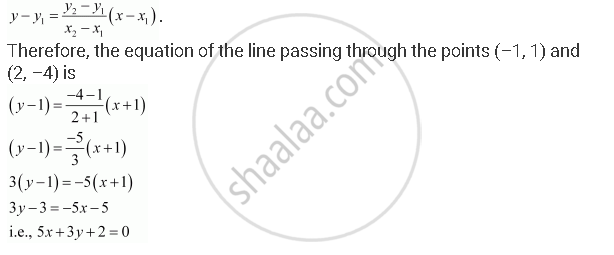CBSE (Arts) Class 11CBSE
Share

# Find the Equation of the Line Which Passes Through the Points (–1, 1) and (2, –4). - CBSE (Arts) Class 11 - Mathematics

ConceptVarious Forms of the Equation of a Line

#### Question

Find the equation of the line which passes through the points (–1, 1) and (2, –4).

#### Solution

It is known that the equation of the line passing through points (x1, y1) and (x2, y2) isIs there an error in this question or solution?

#### Video TutorialsVIEW ALL 

Solution Find the Equation of the Line Which Passes Through the Points (–1, 1) and (2, –4). Concept: Various Forms of the Equation of a Line.
S